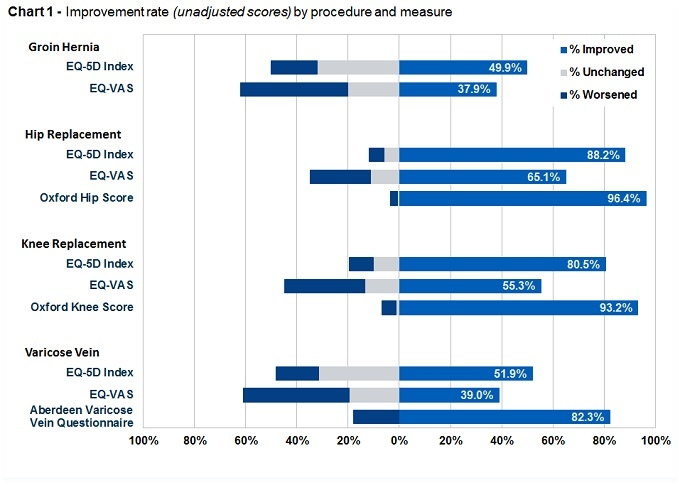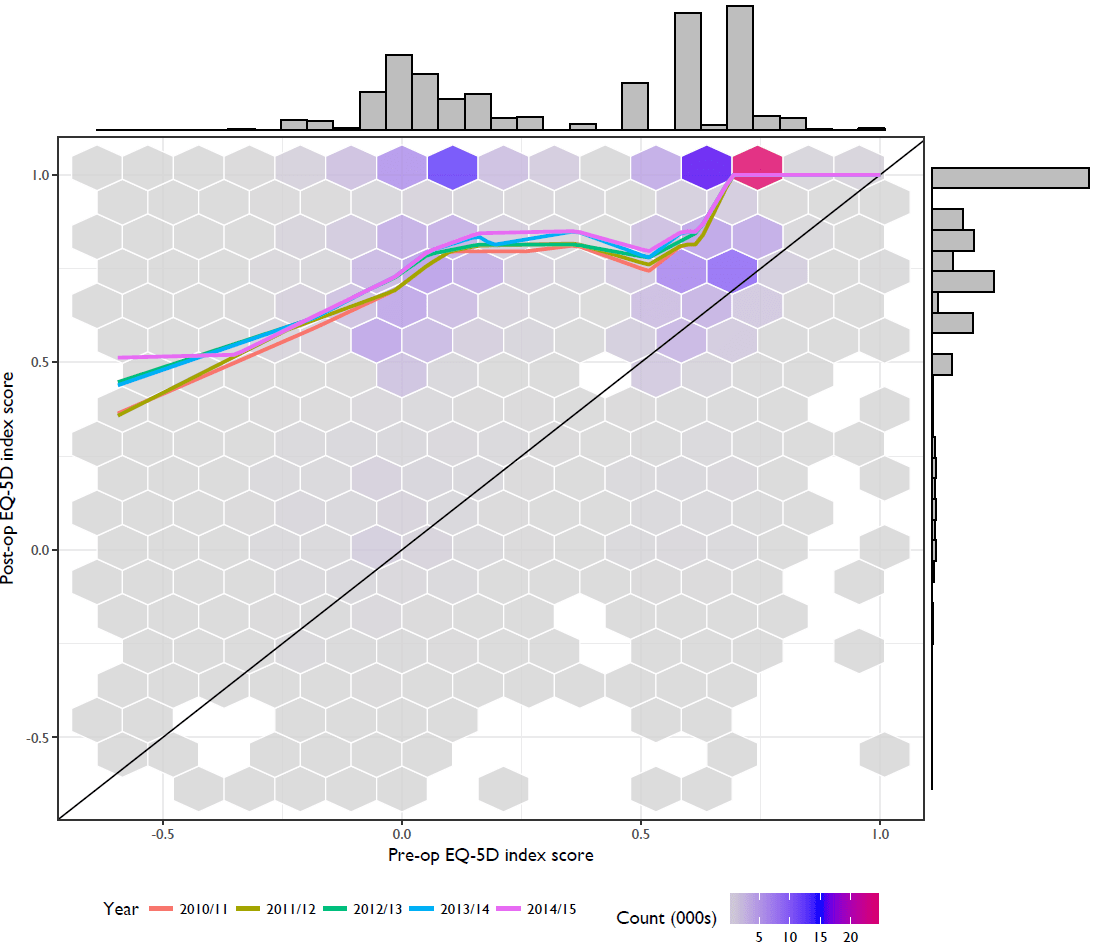# Visualising PROMs data

The patient reported outcomes measures, or PROMs, is a large database with before and after health-related quality of life (HRQoL) measures for a large number of patients undergoing four key conditions: hip replacement, knee replacement, varicose vein surgery and surgery for groin hernia. The outcome measures are the EQ-5D index and visual analogue scale (and a disease-specific measure for three of the interventions). These data also contain the provider of the operation. Being publicly available, these data allow us to look at a range of different questions: what’s the average effect of the surgery on HRQoL? What are the differences between providers in gains to HRQoL or in patient casemix? Great!

The first thing we should always do with new data is to look at it. This might be in an exploratory way to determine the questions to ask of the data or in an analytical way to get an idea of the relationships between variables. Plotting the data communicates more about what’s going on than any table of statistics alone. However, the plots on the NHS Digital website might be accused of being a little uninspired as they collapse a lot of the variation into simple charts that conceal a lot of what’s going on. For example:Finalised Patient Reported Outcome Measures (PROMs) in England – April 2014 to March 2015

So let’s consider other ways of visualising this data. For all these plots a walk through of the code is at the end of this post.

Now, I’m not a regular user of PROMs data, so what I think are the interesting features of the data may not reflect what the data are generally used for. For this, I think the interesting features are:

• The joint distribution of pre- and post-op scores
• The marginal distributions of pre- and post-op scores
• The relationship between pre- and post-op scores over time

We will pool all the data from six years’ worth of PROMs data. This gives us over 200,000 observations. A scatter plot with this information is useless as the density of the points will be very high. A useful alternative is hexagonal binning, which is like a two-dimensional histogram. Hexagonal tiles, which usefully tessellate and are more interesting to look at than squares, can be shaded or coloured with respect to the number of observations in each bin across the support of the joint distribution of pre- and post-op scores (which is [-0.5,1]x[-0.5,1]). We can add the marginal distributions to the axes and then add smoothed trend lines for each year. Since the data are constrained between -0.5 and 1, the mean may not be a very good summary statistic, so we’ll plot a smoothed median trend line for each year. Finally, we’ll add a line on the diagonal. Patients above this line have improved and patients below it deteriorated.

## Hip replacement resultsThere’s a lot going on in the graph, but I think it reveals a number of key points about the data that we wouldn’t have seen from the standard plots on the website:

• There appear to be four clusters of patients:
• Those who were in close to full health prior to the operation and were in ‘perfect’ health (score = 1) after;
• Those who were in close to full health pre-op and who didn’t really improve post-op;
• Those who were in poor health (score close to zero) and made a full recovery;
• Those who were in poor health and who made a partial recovery.
• The median change is an improvement in health.
• The median change improves modestly from year to year for a given pre-op score.
• There are ceiling effects for the EQ-5D.

None of this is news to those who study these data. But this way of presenting the data certainly tells more of a story that the current plots on the website.

## R code

We’re going to consider hip replacement, but the code is easily modified for the other outcomes. Firstly we will take the pre- and post-op score and their difference and pool them into one data frame.

```# df 14/15

df<-df[!is.na(df\$Pre.Op.Q.EQ5D.Index),]
df\$pre<-df\$Pre.Op.Q.EQ5D.Index
df\$post<- df\$Post.Op.Q.EQ5D.Index
df\$diff<- df\$post - df\$pre

df1415 <- df[,c('Provider.Code','pre','post','diff')]

#
# df 13/14

df<-df[!is.na(df\$Pre.Op.Q.EQ5D.Index),]
df\$pre<-df\$Pre.Op.Q.EQ5D.Index
df\$post<- df\$Post.Op.Q.EQ5D.Index
df\$diff<- df\$post - df\$pre

df1314 <- df[,c('Provider.Code','pre','post','diff')]

# df 12/13

df<-df[!is.na(df\$Pre.Op.Q.EQ5D.Index),]
df\$pre<-df\$Pre.Op.Q.EQ5D.Index
df\$post<- df\$Post.Op.Q.EQ5D.Index
df\$diff<- df\$post - df\$pre

df1213 <- df[,c('Provider.Code','pre','post','diff')]

# df 11/12

df\$pre<-df\$Q1_EQ5D_INDEX
df\$post<- df\$Q2_EQ5D_INDEX
df\$diff<- df\$post - df\$pre
names(df)<-'Provider.Code'

df1112 <- df[,c('Provider.Code','pre','post','diff')]

# df 10/11

df\$pre<-df\$Q1_EQ5D_INDEX
df\$post<- df\$Q2_EQ5D_INDEX
df\$diff<- df\$post - df\$pre
names(df)<-'Provider.Code'

df1011 <- df[,c('Provider.Code','pre','post','diff')]

#combine

df1415\$year<-"2014/15"
df1314\$year<-"2013/14"
df1213\$year<-"2012/13"
df1112\$year<-"2011/12"
df1011\$year<-"2010/11"

df<-rbind(df1415,df1314,df1213,df1112,df1011)
write.csv(df,"C:/docs/proms/eq5d.csv")

```

Now, for the plot. We will need the packages ggplot2, ggExtra, and extrafont. The latter package is just to change the plot fonts, not essential, but aesthetically pleasing.

```require(ggplot2)
require(ggExtra)
require(extrafont)
font_import()

p<-ggplot(data=df,aes(x=pre,y=post))+
stat_bin_hex(bins=15,color="white",alpha=0.8)+
geom_abline(intercept=0,slope=1,color="black")+
geom_quantile(aes(color=year),method = "rqss", lambda = 2,quantiles=0.5,size=1)+
mid="blue",high = "red",
breaks=c(5000,10000,15000,20000),labels=c(5,10,15,20))+
theme_bw()+
labs(x="Pre-op EQ-5D index score",y="Post-op EQ-5D index score")+
scale_color_discrete(name="Year")+
theme(legend.position = "bottom",text=element_text(family="Gill Sans MT"))

ggMarginal(p, type = "histogram")```

## Author

•Health economics, statistics, and health services research at the University of Warwick. Also like rock climbing and making noise on the guitar.

This site uses Akismet to reduce spam. Learn how your comment data is processed.